Back to Adaptive Vision Studio website

You are here: Start » Filter Reference » Real

Real

Select a filter from the list below.

IconNameDescription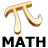AbsoluteRealComputes the absolute value of a number.AddDoublesComputes the sum of two or more doubles.AddDoubles_OfArrayComputes the sum of numbers of an array.AddDoubles_OfLoopComputes the sum of numbers appearing in consecutive iterations.AddRealsComputes the sum of two numbers.AddReals_OfArrayComputes the sum of numbers of an array.AddReals_OfLoopComputes the sum of numbers appearing in consecutive iterations.AverageDoublesReturns the average of two doubles.AverageDoubles_OfArrayReturns the average value of doubles.AverageDoubles_OfArray_OrNilReturns the average value of doubles; returns NIL if the array is empty.AverageDoubles_OfLoopReturns the average of numbers appearing in consecutive iterations.AverageRealsReturns the average of two numbers.AverageReals_OfArrayReturns the average value of reals.AverageReals_OfArray_OrNilReturns the average value of reals; returns NIL if the array is empty.AverageReals_OfLoopReturns the average of numbers appearing in consecutive iterations.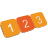CreateRealSequenceCreates an arithmetic sequence of real numbers.DivideDoublesComputes the quotient of two numbers.DivideRealsComputes the quotient of two numbers.DoubleDifferenceComputes the absolute value of two doubles difference.LerpRealsLinearly interpolates between two reals.MaximumDouble_OfArrayReturns the greatest value in an array of double numbers.MaximumDouble_OfArray_OrNilReturns the greatest value in an array of double numbers; returns NIL if the array is empty.MaximumDouble_OfLoopReturns the greatest of numbers appearing in consecutive iterations.MaximumRealReturns the higher of two numbers.MaximumReal_OfArrayReturns the greatest value in an array of real numbers.MaximumReal_OfArray_OrNilReturns the greatest value in an array of real numbers; returns NIL if the array is empty.MaximumReal_OfLoopReturns the greatest of numbers appearing in consecutive iterations.MinimumDouble_OfArrayReturns the smallest value in an array of double numbers.MinimumDouble_OfArray_OrNilReturns the smallest value in an array of double numbers; returns NIL if the array is empty.MinimumDouble_OfLoopReturns the smallest of numbers appearing in consecutive iterations.MinimumRealReturns the lower of two numbers.MinimumReal_OfArrayReturns the smallest value in an array of real numbers.MinimumReal_OfArray_OrNilReturns the smallest value in an array of real numbers; returns NIL if the array is empty.MinimumReal_OfLoopReturns the smallest of numbers appearing in consecutive iterations.MultiplyDoublesComputes the product of double numbers.MultiplyDoubles_OfArrayComputes the product of numbers of an array.MultiplyDoubles_OfLoopComputes the product of numbers appearing in consecutive iterations.MultiplyRealsComputes the product of real numbers.MultiplyReals_OfArrayComputes the product of numbers of an array.MultiplyReals_OfLoopComputes the product of numbers appearing in consecutive iterations.NegateRealComputes the negation of a number.ParseDoubleReturns the double number represented by a string.ParseRealReturns the real number represented by a string.RealDifferenceComputes the absolute value of two numbers difference.RealSequenceDistancesComputes the absolute difference between points in array.RescaleRealApplies linear transformation to real number.SubtractDoublesComputes the difference of two doubles.SubtractRealsComputes the difference of two numbers.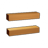TestDoubleEqualToChecks whether two doubles are equal.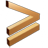TestDoubleGreaterOrEqualCompares two doubles with the >= operator.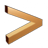TestDoubleGreaterThanCompares two doubles with the > operator.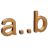TestDoubleInRangeChecks whether a double value is in the specified range.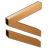TestDoubleLessOrEqualCompares two doubles with the <= operator.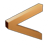TestDoubleLessThanCompares two doubles with the < operator.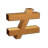TestDoubleUnequalToChecks whether two doubles are unequal.TestRealEqualToChecks whether two reals are equal.TestRealGreaterOrEqualCompares two reals with the >= operator.TestRealGreaterThanCompares two reals with the > operator.TestRealInRangeChecks whether a real value is in the specified range.TestRealLessOrEqualCompares two reals with the <= operator.TestRealLessThanCompares two reals with the < operator.TestRealUnequalToChecks whether two reals are unequal.